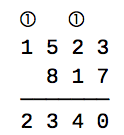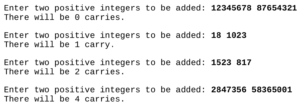# Carry Counts

Hello all!

It’s been a while since I’ve been able to post, but I wanted to cover another Bloomsburg problem from 2018 for my programming club.  The problem description is this:

When calculating the sum of two numbers by hand, we first add the digits in the 1’s position, and if the result overflows (i.e., is greater than 9) then we carry the leftmost 1 in the result to the 10’s position. This process is repeated at the 10’s position, then at the 100’s position, and so on. For example, if we are calculating 1523 + 817 by hand, we write:In this case, there are two carries (shown in circles).

Write a program that prompts the user for two positive integers and outputs the number of carries that would occur when performing addition by hand.

The following test cases illustrate the required I/O format.We’ll begin this problem by defining our variables and taking in some input.  Our input will be the two integers we will be adding, and then we will be putting them into a list.

carries = 0
int1 = int(raw_input("Enter two positive integers to be added: "))
int2 = int(raw_input())
ints = [int1, int2]

Next up we need to define our algorithm we will be using.   For this problem we will be using a new function called zfill(). The zfill() function fills a string up with zeroes on the left side until a specific length is reached.  We’ll also be using the .max() method to find the maximum value of the list, and then run the len() method on it to get the max length.  Doing this we can fill up both integers until they are the same length, allowing me to then work backwords through them, adding each value from right to left and counting the carries for each value.

length = len(max(ints))
add2 = int2.zfill(length)

Then we’ll use a for loop to loop through the length of the integers, moving from right to left and adding each value.  If the summed value is greater than 9, then the value will have a carry.  We also need to create a variable called extra, which I will use as the actual carry, because in a case like 89+11 the carry from the ones value causes the tens value to also increase by one, so we must account for it.  Otherwise if the summed value is less than or equal to nine, we do nothing and set extra equal to zero.

for i in range(length):
extra = 1
carries += 1
extra = 0

After we’ve iterated through the integers, we just need to check how many carries there were and print our statements accordingly for grammatical correctness.

if carries == 1:
print("There will be 1 carry.")
elif carries <= 0:
print("There will be no carries.")
else:
print("There will be ", carries, " carries")

And now we have a working solution to Bloomsburg 2018 Problem 3, Carry Counts.  I hope this helps. 🙂

Thanks for reading and have a wonderful day!
~ Corbin